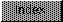```

NAME
SPMul -- Multiply two floating point numbers.

SYNOPSIS
fnum3 = SPMul(fnum1, fnum2)
D0            D1     D0

float SPMul(float fnum1, float fnum2);

FUNCTION
Accepts two floating point numbers and returns the arithmetic
multiplication of said numbers.

INPUTS
fnum1   - floating point number
fnum2   - floating point number

RESULT
fnum3   - floating point number

BUGS
None